# PointWithDescription¶

class PointWithDescription(*args)

Collection of real values with a description for each component.

Available constructors:

PointWithDescription(size=0, value=0.0)

PointWithDescription(sequence)

Parameters:
sizeint,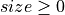Size of the vector.

valuefloat

Value set to the size elements.

sequencesequence of pair (string, float)

Components of the vector.

Examples

>>> import openturns as ot


Use the first constructor:

>>> print(ot.PointWithDescription(2))
[ : 0,  : 0]
>>> vector = ot.PointWithDescription(2, 3.0)
>>> print(vector)
[ : 3,  : 3]
>>> vector.setDescription(['c1', 'c2'])
>>> print(vector)
[c1 : 3, c2 : 3]


Use the second constructor:

>>> vector = ot.PointWithDescription([('C1', 2.0), ('C2', 3.0), ('C3', 4.5)])
>>> print(vector)
[C1 : 2, C2 : 3, C3 : 4.5]
>>> print(vector.getDescription())
[C1,C2,C3]


Use some functionalities:

>>> vector = 7.1
>>> print(vector)
[C1 : 2, C2 : 7.1, C3 : 4.5]
>>> print(vector)
[C1 : 2, C2 : 7.1, C3 : 4.5,  : 6.2]


Methods

 add(*args) Append a component (in-place). at(*args) Access to an element of the collection. Reset the collection to zero dimension. dot(rhs) Compute the scalar product. find(val) Find the index of a given value. Accessor to the object's name. Accessor to the componentwise description. Accessor to the vector's dimension. Accessor to the object's id. Accessor to the object's name. Accessor to the object's shadowed id. Accessor to the vector's dimension (or size). Accessor to the object's visibility state. Test if the object is named. Test if the object has a distinguishable name. Check if the components are in decreasing order. Tell if the collection is empty. Check if the components are in increasing order. Check if the components are in nonincreasing or nondecreasing order. Check if the components are in nondecreasing order. Check if the components are in nonincreasing order. Compute the Euclidean (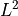) norm. Compute the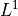norm. Compute the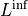norm. Compute the squared Euclidean norm. Compute the normalized vector with respect to its Euclidean norm. Compute the normalized vector with respect to its squared Euclidean norm. resize(newSize) Change the size of the collection. select(marginalIndices) Selection from indices. setDescription(description) Accessor to the componentwise description. setName(name) Accessor to the object's name. Accessor to the object's shadowed id. setVisibility(visible) Accessor to the object's visibility state.
 clean
__init__(*args)

Append a component (in-place).

Parameters:
valuetype depends on the type of the collection.

The component to append.

Examples

>>> import openturns as ot
>>> x = ot.Point(2)
>>> print(x)
[0,0,1]

at(*args)

Parameters:
indexpositive int

Position of the element to access.

Returns:
elementtype depends on the type of the collection

Element of the collection at the position index.

clear()

Reset the collection to zero dimension.

Examples

>>> import openturns as ot
>>> x = ot.Point(2)
>>> x.clear()
>>> x
class=Point name=Unnamed dimension=0 values=[]

dot(rhs)

Compute the scalar product.

Parameters:
pointsequence of float

Scalar product second argument

Returns:
dotfloat

Scalar product

Examples

>>> import openturns as ot
>>> x = ot.Point([1.0, 2.0, 3.0])
>>> prod = x.dot([4, 5, 6])

find(val)

Find the index of a given value.

Parameters:
valcollection value type

The value to find

Returns:
indexint

The index of the first occurrence of the value, or the size of the container if not found. When several values match, only the first index is returned.

getClassName()

Accessor to the object’s name.

Returns:
class_namestr

The object class name (object.__class__.__name__).

getDescription()

Accessor to the componentwise description.

Returns:
descriptionDescription

Description of the components.

getDimension()

Accessor to the vector’s dimension.

Returns:
nint

The number of components in the vector.

getId()

Accessor to the object’s id.

Returns:
idint

Internal unique identifier.

getName()

Accessor to the object’s name.

Returns:
namestr

The name of the object.

Accessor to the object’s shadowed id.

Returns:
idint

Internal unique identifier.

getSize()

Accessor to the vector’s dimension (or size).

Returns:
nint

The number of components in the vector.

getVisibility()

Accessor to the object’s visibility state.

Returns:
visiblebool

Visibility flag.

hasName()

Test if the object is named.

Returns:
hasNamebool

True if the name is not empty.

hasVisibleName()

Test if the object has a distinguishable name.

Returns:
hasVisibleNamebool

True if the name is not empty and not the default one.

isDecreasing()

Check if the components are in decreasing order.

Examples

>>> import openturns as ot
>>> x = ot.Point([3.0, 2.0, 1.0])
>>> x.isDecreasing()
True
>>> x = ot.Point([3.0, 3.0, 1.0])
>>> x.isDecreasing()
False
>>> x = ot.Point([1.0, 3.0, 2.0])
>>> x.isIncreasing()
False

isEmpty()

Tell if the collection is empty.

Returns:
isEmptybool

True if there is no element in the collection.

Examples

>>> import openturns as ot
>>> x = ot.Point(2)
>>> x.isEmpty()
False
>>> x.clear()
>>> x.isEmpty()
True

isIncreasing()

Check if the components are in increasing order.

Examples

>>> import openturns as ot
>>> x = ot.Point([1.0, 2.0, 3.0])
>>> x.isIncreasing()
True
>>> x = ot.Point([1.0, 1.0, 3.0])
>>> x.isIncreasing()
False
>>> x = ot.Point([1.0, 3.0, 2.0])
>>> x.isIncreasing()
False

isMonotonic()

Check if the components are in nonincreasing or nondecreasing order.

Examples

>>> import openturns as ot
>>> x = ot.Point([1.0, 2.0, 3.0])
>>> x.isMonotonic()
True
>>> x = ot.Point([2.0, 2.0, 1.0])
>>> x.isMonotonic()
True
>>> x = ot.Point([1.0, 3.0, 2.0])
>>> x.isMonotonic()
False

isNonDecreasing()

Check if the components are in nondecreasing order.

Examples

>>> import openturns as ot
>>> x = ot.Point([1.0, 2.0, 3.0])
>>> x.isNonDecreasing()
True
>>> x = ot.Point([1.0, 1.0, 3.0])
>>> x.isNonDecreasing()
True
>>> x = ot.Point([1.0, 3.0, 2.0])
>>> x.isNonDecreasing()
False

isNonIncreasing()

Check if the components are in nonincreasing order.

Examples

>>> import openturns as ot
>>> x = ot.Point([3.0, 2.0, 1.0])
>>> x.isNonIncreasing()
True
>>> x = ot.Point([3.0, 3.0, 1.0])
>>> x.isNonIncreasing()
True
>>> x = ot.Point([1.0, 3.0, 2.0])
>>> x.isNonIncreasing()
False

norm()

Compute the Euclidean () norm.

The Euclidean () norm of a vector is defined as: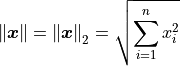Returns:
normfloat

The vector’s Euclidean norm.

Examples

>>> import openturns as ot
>>> x = ot.Point([1.0, 2.0, 3.0])
>>> x.norm()
3.741657...

norm1()

Compute thenorm.

Thenorm of a vector is defined as: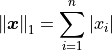Returns:
normfloat

The vector’snorm.

Examples

>>> import openturns as ot
>>> x = ot.Point([1.0, 2.0, 3.0])
>>> x.norm1()
6.0

normInf()

Compute thenorm.

Thenorm of a vector is defined as: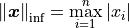Returns:
normfloat

The vector’snorm.

Examples

>>> import openturns as ot
>>> x = ot.Point([1.0, 2.0, 3.0])
>>> x.normInf()
3.0

normSquare()

Compute the squared Euclidean norm.

Returns:
normfloat

The vector’s squared Euclidean norm.

Examples

>>> import openturns as ot
>>> x = ot.Point([1.0, 2.0, 3.0])
>>> x.normSquare()
14.0

normalize()

Compute the normalized vector with respect to its Euclidean norm.

Returns:
normalized_vectorPoint

The normalized vector with respect to its Euclidean norm.

Raises:
RuntimeErrorIf the Euclidean norm is zero.

Examples

>>> import openturns as ot
>>> x = ot.Point([1.0, 2.0, 3.0])
>>> print(x.normalize())
[0.267261,0.534522,0.801784]

normalizeSquare()

Compute the normalized vector with respect to its squared Euclidean norm.

Returns:
normalized_vectornormalized_vectorPoint

The normalized vector with respect to its squared Euclidean norm.

Raises:
RuntimeErrorIf the squared Euclidean norm is zero.

Examples

>>> import openturns as ot
>>> x = ot.Point([1.0, 2.0, 3.0])
>>> print(x.normalizeSquare())
[0.0714286,0.285714,0.642857]

resize(newSize)

Change the size of the collection.

Parameters:
newSizepositive int

New size of the collection.

Notes

If the new size is smaller than the older one, the last elements are thrown away, else the new elements are set to the default value of the element type.

Examples

>>> import openturns as ot
>>> x = ot.Point(2, 4)
>>> print(x)
[4,4]
>>> x.resize(1)
>>> print(x)

>>> x.resize(4)
>>> print(x)
[4,0,0,0]

select(marginalIndices)

Selection from indices.

Parameters:
indicessequence of int

Indices to select

Returns:
collsequence

Sub-collection of values at the selection indices.

setDescription(description)

Accessor to the componentwise description.

Parameters:
descriptionsequence of str

Description of the components.

setName(name)

Accessor to the object’s name.

Parameters:
namestr

The name of the object.

Accessor to the object’s shadowed id.

Parameters:
idint

Internal unique identifier.

setVisibility(visible)

Accessor to the object’s visibility state.

Parameters:
visiblebool

Visibility flag.

## Examples using the class¶Analyse the central tendency of a cantilever beam

Analyse the central tendency of a cantilever beam